Updating search results...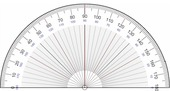# Finite Mathematics

Systems of linear equations, matrices, and matrix algebra; linear inequalities; linear programming; counting techniques: permutations and combinations; probability; basic concepts in financial mathematics (annuities included); and an introduction to statistics.

6 affiliated resources

# Search Resources

View
Selected filters: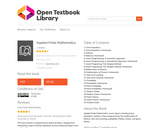Unrestricted Use
CC BY
Rating
0.0 stars

The text covered a comprehensive extent of topics; ranging from introductory topics of linear equations to more advanced topics such as Game Theory.

Subject:
Mathematics
Material Type:
Textbook
Author:
De Anza College Cupertino
Rupinder Sekhon
03/15/2019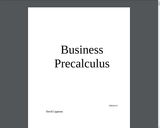Conditional Remix & Share Permitted
CC BY-SA
Rating
0.0 stars

Chapter 1: Functions and Lines
Chapter 2: Systems of Equations and Matrices
Chapter 3: Linear Programming
Chapter 4: Polynomial and Rational Functions
Chapter 5: Exponential and Logarithmic Functions
Chapter 6: Finance
Chapter 7: Sets
Chapter 8: Probability

Subject:
Mathematics
Material Type:
Textbook
Author:
David Lippman
08/04/2020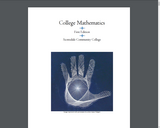Conditional Remix & Share Permitted
CC BY-NC-SA
Rating
0.0 stars

Chapter 1: Fundamentals of Geometry
Chapter 2 – Measurement and Dimensional Analysis
Chapter 3: Linear Functions
Chapter 4: Percentages
Chapter 5: Savings
Chapter 6: Annuities and Loans
Chapter 7: Sets and Venn Diagrams
Chapter 8 – Introduction to Probability
Chapter 9 – Probability and Counting
Chapter 10 - Statistics
Chapter 11 – Describing Data
Chapter 12 – The Normal Distribution

Subject:
Mathematics
Material Type:
Textbook
Author:
Carla Stroud
Jenifer Bohart
Tracey Haynie
08/04/2020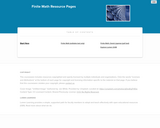Educational Use
Rating
0.0 stars

Mathematics

Subject:
Mathematics
Material Type:
Full Course
Textbook
Provider:
Lumen Learning
Provider Set:
Candela Courseware
04/25/2019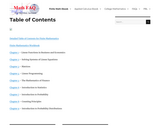Only Sharing Permitted
CC BY-NC-ND
Rating
0.0 stars

Chapter 1 – Linear Functions in Business and Economics
Chapter 2 – Solving Systems of Linear Equations
Chapter 3 – Matrices
Chapter 4 – Linear Programming
Chapter 5 – The Mathematics of Finance
Chapter 6 – Introduction to Statistics
Chapter 7 – Introduction to Probability
Chapter 8 – Counting Principles
Chapter 9 – Introduction to Probability Distributions

Includes a Finite Mathematics Workbook: https://math-faq.com/wp/finite-mathematics-workbook/ . In each workbook, you will find key terms, a short summary, and guided problems for each section in the ebook. Accompanying each guided example is a practice problem for students to complete. Answers to the practice problems are available at the end of each chapter’s workbook.

Subject:
Mathematics
Material Type:
Activity/Lab
Textbook
Author:
Pearson Education
06/18/2020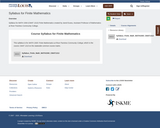Unrestricted Use
CC BY
Rating
0.0 stars

Syllabus for MATH 1500 (CMAT 1313) Finite Mathematics created by Jared Eusea, Assistant Professor of Mathematics at River Parishes Community College.

Subject:
Mathematics
Material Type:
Syllabus
Author:
Jared Eusea